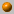##### Personal tools
You are here: Home Computational Geometry - Fall 2017/2018
« February 2023 »
February
SuMoTuWeThFrSa
1234
567891011
12131415161718
19202122232425
262728

# Computational Geometry - Fall 2017/2018

0368-3173-01

Monday, 16:00-19:00, Melamed 006

Instructor: Dan Halperin , danha@post
Office hours: by appointment

TA: Michal Kleinbort, balasmic AT post.tau.ac.il
Office hours: by appointment

Maibox no. 312, Schriebr, 1st floor

The course covers fundamental algorithms for solving geometric problems such as computing convex hulls, intersection of line segments, Voronoi diagrams, polygon triangulation, and linear programming in low dimensional space. We will also discuss several applications of geometric algorithms to solving problems in robotics, GIS (geographic information systems), computer graphics, and more.

### Prerequisites:

Data Structures, Algorithms and knowledge of C++ or willingness to learn the language

### Slides:

I will often use slides that accompany the main textbook of the course.The slides are by Marc van Kreveld and they can be found

at the following site: Geometric Algorithms

### Bibliography:

The main textbook of the course is:

Computational Geometry: Algorithms and Applications (CGAA), 2nd or 3rd edition by M. de Berg, M. van Kreveld, M. Overmars, and O. Schwarzkopf.

A bibliographic list for the course

The standard assignments will account for 10% of the final grade, in case they improve the final grade.

The grade in the final exam, to be held on Wednesday, February 14th, 2018, will account for the remaining 90% of the grade in the course.

Exam(ple), pdf file

Although the assignment grades will be used only for improving the final grade, submission of the assignments is mandatory and a prerequisite for taking the exam.

About halfway through the course, we will present a programming project.

We encourage you to submit the programming project as well. This is not mandatory.

If however you do submit it, then your grade breakdown will be 10% the standard assignments,
15% the programming project, and 75% the final exam. Here as well,
the assignments grades and project grade will be counted toward the final grade only if they improve it.

There is an option to do the project in pairs, in which case its weight will be only 10% for each student of the pair instead of 15%.

The assignments will appear here.

Assignment no. 1 file , Part I due: November 13th, 2017. Part II due: November 20th, 2017.
Submission guidelines

Assignment no. 2 file , due: December 4th, 2017.

Assignment no. 3 file , due: December 25th, 2017.

Programming assignment (pdf file), due: January 11th, 2018, 23:55.

Assignment no. 4 file , due: January 21th, 2018 (updated due date).

Assignment no. 5 file , not for submission.

### Course Summary:

Below you'll find a very brief summary of what was covered in class during the semester.
This should not be taken as a complete description of the course's contents. "Slides X" refers to the relevant set of slides from Geometric Algorithms.

For an outline of the course, see for example, the course summary in the 2010 computational geometry course.23.10.17
What is Computational Geometry?
Course outline, motivation and techniques. A slow convex hull algorithm. Orientation (Side-of-line) test. Graham's O(n log n) algorithm (Chapter 1 in CGAA). Lower bound.    (Slides 1.)30.10.17
Gift-wrapping algorithm for computing the convex hull.
Output sensitive algorithm to compute the intersections of line segments: Bentley Ottmann's plane sweep; handling degeneracies (CGAA, Chapter 2). (Slides 2a.)6.11.17
The doubly-connected edge list (DCEL). Map overlay and applications (CGAA, Chapter 2).
The Douglas-Peucker algorithm for line simplification.13.11.17
Polygon triangulation and art gallery problems - Part I: combinatorial bounds and properties (O'Rourke's book CG in C, Chapters 1 and 2, and in CGAA, Chapter 3). Part II: Triangulating a monotone polygon (CGAA, Chapter 3) and decomposing a polygon into monotone polygons via trapezoidal decomposition (O'Rourke's book, Chapter 2).20.11.17
We have shown that \Omega(n^{3/2} guards are sometimes needed to guard a polyhedron with n vertices. The construction is due to Seidel and its presentation is taken from               the book Art Gallery Theorems and Algorithms by J. O'Rourke.
The casting problem. Half plane intersection. Introduction to Linear Programming (CGAA, Chapter 4). (Slides 4a)27.11.17
Linear programming in 2D continued: the unbounded case (CGAA, Chapter 4).
Linear-time solution to the casting problem (paper).4.12.17
Linear programming in 3D (CGAA, Chapter 4).
Orthogonal range searching, kd-trees (CGAA, Chapter 5).11.12.17
Orthogonal range searching, range trees, handling degeneracies through composite numbers (CGAA, Chapter 5).
A divide-and-conquer algorithm for computing the intersection of half-planes.18.12.17
Introduction to CGAL [pdf]
Recitation: NN search using kd-trees, LP.25.12.17
Trapezoidal maps and planar point location (CGAA, Chapter 6).8.1.18
Voronoi diagrams of points in the plane: structure, complexity, and Fortune's algorithm (CGAA, Chapter 7). [partial proof of lemma]
Legal triangulations and Delaunay triangulations (CGAA, Chapter 9).15.1.18
Point-Line duality, arrangements of lines in the plane, the zone theorem and incremental construction of the arrangement (CGAA, Chapter 8).                                                                 The minimum area triangle problem (CGAL Arrangements and Their Applications, Section 4.2.1).
The lifting transform.
The connection between convex hulls, envelopes, intersection of half-spaces, Voronoi diagrams and Delaunay triangualtions (partly covered in CGAA, Sections 11.4 and 11.5).

### Forum:

We will use piazza platform for our forum discussions.